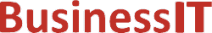O nás     Inzerce     KontaktSpolehlivé informace o IT již od roku 2011[Linux manuál]

# ilogb, ilogbf, ilogbl: vrátit nezaujatý exponent

Originální popis anglicky: ilogb, ilogbf, ilogbl - return an unbiased exponent

Návod, kniha: POSIX Programmer's Manual

## STRUČNĚ

#include <math.h>

int ilogb(double x);

int ilogbf(float x);

int ilogbl(long double x);

## POPIS / INSTRUKCE

These functions shall return the exponent part of their argument x. Formally, the return value is the integral part of log_r|x| as a signed integral value, for non-zero x, where r is the radix of the machine's floating-point arithmetic, which is the value of FLT_RADIX defined in <float.h>.
An application wishing to check for error situations should set errno to zero and call feclearexcept(FE_ALL_EXCEPT) before calling these functions. On return, if errno is non-zero or fetestexcept(FE_INVALID | FE_DIVBYZERO | FE_OVERFLOW | FE_UNDERFLOW) is non-zero, an error has occurred.

## NÁVRATOVÁ HODNOTA

Upon successful completion, these functions shall return the exponent part of x as a signed integer value. They are equivalent to calling the corresponding logb() function and casting the returned value to type int.
If x is 0,  a domain error shall occur, and the value FP_ILOGB0 shall be returned.
If x is ±Inf,  a domain error shall occur, and the value {INT_MAX} shall be returned.
If x is a NaN,  a domain error shall occur, and the value FP_ILOGBNAN shall be returned.
If the correct value is greater than {INT_MAX}, {INT_MAX} shall be returned and a domain error shall occur.
If the correct value is less than {INT_MIN}, {INT_MIN} shall be returned and a domain error shall occur.

## CHYBY / ERRORY

These functions shall fail if:
Domain Error
The x argument is zero, NaN, or ±Inf, or the correct value is not representable as an integer.
If the integer expression (math_errhandling & MATH_ERRNO) is non-zero, then errno shall be set to [EDOM]. If the integer expression (math_errhandling & MATH_ERREXCEPT) is non-zero, then the invalid floating-point exception shall be raised.

The following sections are informative.

None.

## APPLICATION USAGE

On error, the expressions (math_errhandling & MATH_ERRNO) and (math_errhandling & MATH_ERREXCEPT) are independent of each other, but at least one of them must be non-zero.

## RATIONALE

The errors come from taking the expected floating-point value and converting it to int, which is an invalid operation in IEEE Std 754-1985 (since overflow, infinity, and NaN are not representable in a type int), so should be a domain error.
There are no known implementations that overflow. For overflow to happen, {INT_MAX} must be less than LDBL_MAX_EXP* log2(FLT_RADIX) or {INT_MIN} must be greater than LDBL_MIN_EXP* log2(FLT_RADIX) if subnormals are not supported, or {INT_MIN} must be greater than (LDBL_MIN_EXP-LDBL_MANT_DIG)* log2(FLT_RADIX) if subnormals are supported.

None.

## SOUVISEJÍCÍ

feclearexcept() , fetestexcept() , logb() , scalb() , the Base Definitions volume of IEEE Std 1003.1-2001, Section 4.18, Treatment of Error Conditions for Mathematical Functions, <float.h>, <math.h> Portions of this text are reprinted and reproduced in electronic form from IEEE Std 1003.1, 2003 Edition, Standard for Information Technology -- Portable Operating System Interface (POSIX), The Open Group Base Specifications Issue 6, Copyright (C) 2001-2003 by the Institute of Electrical and Electronics Engineers, Inc and The Open Group. In the event of any discrepancy between this version and the original IEEE and The Open Group Standard, the original IEEE and The Open Group Standard is the referee document. The original Standard can be obtained online at http://www.opengroup.org/unix/online.html .
 2003 IEEE/The Open Group
©2011-2020 BusinessIT.cz, ISSN 1805-0522 | Názvy použité v textech mohou být ochrannými známkami příslušných vlastníků.
Provozovatel: Bispiral, s.r.o., kontakt: BusinessIT(at)Bispiral.com | Inzerce: Best Online Media, s.r.o., zuzana@online-media.cz
O vydavateli | Pravidla webu BusinessIT.cz a ochrana soukromí | pg(9142)